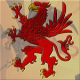## Species definition clarification and exercises

[This is the fourth in a series of posts about combinatorial species. Previous posts: And now, back to your regularly scheduled combinatorial species; Decomposing data structures; Combinatorial species definition.]

In my previous post I neglected to mention something quite crucial, namely, that (at least for now) we are only talking about finite sets of labels and finite sets of structures. That is, we do not consider structures with infinitely many labels, and given a particular finite set of labels, there must be only a finite number of structures with those labels. The category$\mathbb{B}$ is the category of finite sets and bijections, not of all sets as I said previously.

Of course, in practice we do want to think about infinite sets of structures and labels, especially in relation to a non-strict language like Haskell! But the theory was invented by mathematicians interested in counting things. I do intend to explore ways to extend the theory to encompass infinite structures, but for now we’ll stick to the finite.

Before moving on to talk about the algebraic approach to species, I also want to point out a few simple implications of the formal definition. Instead of spelling them out in detail, I will pose them as exercises: you can either take them on faith, or try working through the exercises to deepen your understanding.

1. Let$[n]$ denote the set$\{0, \dots, n-1\}$1, and write$F[n]$ as an abbreviation for$F[[n]]$, i.e. the application of the species$F$ to the label set$[n]$. Show that given$F[n]$ we can determine$F[U]$ for any$U$ with$|U| = n$.

(This shows that “size is all that matters”: in some sense species are really indexed not by sets of labels but by size.)

1. [BLL2 Exercise 1.1.2] Show that we get an equivalent definition if we take species to be functors from$\mathbb{B}$ to$\mathbf{FinSet}$ (the category of finite sets and (total) functions) instead of endofunctors on$\mathbb{B}$.

(Apparently one can generalize the notion of species by replacing$\mathbf{FinSet}$ with other categories, but at present I am not sure of the implications.)

1. The species literature uses$\{1, \dots, n\}$, but (as every good computer scientist knows) counting ought to begin at zero.

2. I will use “BLL” to refer to Bergeron, Labelle, and Leroux, Combinatorial Species and Tree-Like Structures (see the references at the end of the previous post for the full citation).1.Colin Adams says: